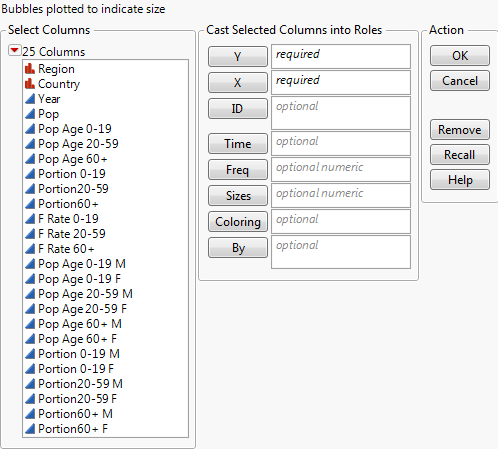Essential Graphing > Bubble Plots > Launch the Bubble Plot Platform
Publication date: 07/30/2020

# Launch the Bubble Plot Platform

Launch the Bubble Plot platform by selecting Graph > Bubble Plot.

Figure 5.4 The Bubble Plot Launch WindowY, X

The Y and X columns become the y and x coordinates of the bubbles in the plot. These values can be continuous or categorical (nominal or ordinal).

ID

ID variables identify rows that should be aggregated and shown as a single bubble. The default coordinates of each bubble are the averaged x and y values, and the default size of each bubble is the sum of the sizes of all aggregated members. See Specifying Two ID Variables.

Time

Maintains separate coordinates, sizes, and colors for each unique time period. The bubble plot shows these values for a single time period. For example, if the Time column contains years, the bubble plot is updated to show data by each year. See Specifying a Time Variable.

Freq

Weights computations when aggregating bubbles using an ID variable.

Note: Negative frequency values are ignored.

Sizes

Controls the size of the bubbles. The area of the bubbles is proportional to the Size value. There is a minimum bubble size, to keep bubbles visible, even if the size value is zero. If Size is left blank, the default bubble size is proportional to the number of rows in that combination of Time and ID.

Coloring

Colors the bubbles according to the selected variable. If the selected variable is categorical (nominal or ordinal), each category is assigned a unique color. If the selected variable is continuous, a gradient of colors is used. You can set a preference for a color theme by selecting File > Preferences > Graphs and making a selection in the Color Themes panel.

By

Place a column here to produce a separate bubble plot for each level of the variable.

After you click OK, the Bubble Plot report window appears.